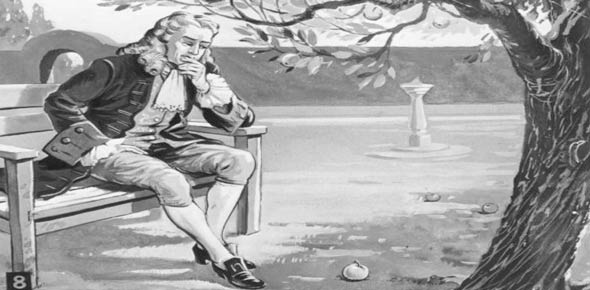Newton's Law's Of Motion

14 Questions | Total Attempts: 229SettingsOnce you have completed this quiz with a score of 90% or more, get a teacher's signature to move on to "Types of Forces" quiz.

• 1.
According to Newton's first law, an object with a net force of zero acting on it will
• A.

Stay at rest

• B.

Move with constant velocity

• C.

Accelerate

• D.

Either A or B

• 2.
The size of a force depends on an object's ________ and ________.
• A.

Gravity and velocity

• B.

Speed and direction

• C.

Mass and acceleration

• D.

Time and distance

• E.

Speed and velocity

• 3.
The relationship between mass and inertia is:
• A.

The larger the mass the smaller the inertia

• B.

The larger the mass the larger the inertia

• C.

Smaller the mass the larger the inertia

• D.

Smaller the mass the smaller the inertia

• 4.
A rock is propelled along a straight line in deep space by a constant force. If the force on the rocket decreases by half, its acceleration
• A.

Stays the same

• B.

Decreases by half

• C.

Triples

• D.

Doubles

• 5.
A softball player hits a ball with a bat. The action force is the impact of the bat against the ball. The reaction this force is the
• A.

Weight of the ball

• B.

Air resistance of the ball

• C.

Force of the ball against the bat

• D.

The force of the grip of the player and the bat

• 6.
An unfortunate bug splatters on your windshield while you are driving your car. Compared to the force of the car on the bug, the force of the bug on the car is
• A.

Less and in the opposite direction

• B.

Greater and in the same direction

• C.

Equal and in opposite direction

• D.

Equal and in same direction

• 7.
In Question #6 above, does the bug or the car have the greater acceleration?
• A.

They both have the same acceleration

• B.

The car has the greater acceleration

• C.

The bug has the greater acceleration

• 8.
When a gun is fired, both the bullet and the gun undergo acceleration. The bullet's acceleration is
• A.

More than the gun's acceleration because it has less mass

• B.

More than the gun's acceleration because it receives more force

• C.

Less than the gun's acceleration because it receives less force

• D.

Less than the gun's acceleration because it has less mass

• 9.
Newton's First Law of Motion states that objects in motion will_________________.
• A.

Stay in motion unless acted upon by an outside force.

• B.

Eventually slow down. Everything comes to rest eventually.

• C.

Speed up.

• D.

Stay in motion because there is a constant force pushing it.

• E.

Stop immediately.

• 10.
If a ball is at rest on top of a ramp what is the sum of all the forces acting on the ball?
• A.

0, there are no forces acting on the ball.

• B.

9.8 N since that is the force due to gravity.

• C.

We cannot determine the sum of the forces based on the given information.

• D.

0, there are multiple forces acting on the ball but they all cancel each other out.

• 11.
If a ball were pushed down a ramp in outer space the ball would:
• A.

Speed up since gravity is acting on it.

• B.

Speed up for awhile but then it would slow down.

• C.

Speed up while you were pushing it, and then it would maintain its velocity.

• D.

Slow down immediately since there is no gravity in outerspace.

• 12.
If forces on an object are unbalanced it will
• A.

Stay in one place

• B.

Move upwards

• C.

Move in the direction of the greater force

• D.

Move in the direction of the smaller force

• 13.
While playing a kick ball game, you can kick long home runs with the rubber ball you are using. Unfortunately, the ball pops. Luckily, someone has a soccer ball. You notice that when you kick the heavier soccer ball, the ball does not travel as quickly and you can only make it to second base. This is because
• A.

Objects in motion remain in motion unless a force is applied.

• B.

Objects at rest remain at rest unless a force is applied.

• C.

The same force accelerates objects with less mass more than objects with more mass.

• D.

For every action there is an equal and opposite reaction.

• 14.
A sail boat is sailing along the lake. Which of the following describes the force pair that helps move the sail boat?            Action Force         :          Reaction Force
• A.

Air Resistance on wind : Wind on air resistance

• B.

Wind blowing on the water : Water blowing on wind

• C.

Wind pushing on sail : Sail pushing on wind

• D.

Sail pushing on wind : Wind pushing on sail

Related TopicsBack to top